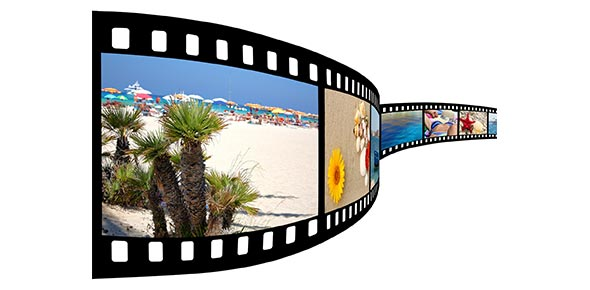# Lifting The Lid: How Computers Work

30 QuestionsSettingsWe watched this video in class together - you can watch the whole thing again before answering these questions or stop and start the video and answer the questions as you go. Click here to play the video: Lifting the lid

Related Topics
• 1.
A general term for devices that plug into a computer is:
• A.

Software

• B.

Hardware

• C.

Peripherals

• D.

Programs

• 2.
What does the cooling fan do?
• A.

Cool the CPU

• B.

Cool the screen

• C.

Keep the person using the computer cool

• D.

Keep dust away from the CPU

• 3.
CPU stands for central __________ unit (One word)
• 4.
What does the computer use RAM for?
• A.

To store programs and files when it is turned off

• B.

To store instructions and data while it is running

• C.

To cool the CPU

• D.

To read data on a CD-ROM

• 5.
BIOS stands for:
• A.

Basic Inside Outside System

• B.

Basic Input Output System

• C.

• D.

Biometric Input Output System

• 6.
What tells a computer what to do when it starts up?
• A.

RAM

• B.

ROM

• C.

CPU

• D.

BIOS

• 7.
What does ROM stand for? ____ ____ ______  (Three words)
• 8.
Where are programs and files stored permanently?
• A.

Floppy Disk Drive

• B.

CD-Rom

• C.

Hard Disk Drive

• D.

CPU

• 9.
Which of these is NOT a card that you would find in a computer?
• A.

Modem

• B.

Network

• C.

Video

• D.

Dance

• E.

Sound

• 10.
A keyboard is an output device.
• A.

True

• B.

False

• 11.
A monitor is an output device.
• A.

True

• B.

False

• 12.
Speakers are an output device.
• A.

True

• B.

False

• 13.
A mouse is an input device.
• A.

True

• B.

False

• 14.
How does the CD or DVD drive read the information on the disk?
• A.

• B.

Laser

• C.

Camera

• D.

Scanner

• 15.
What number is represented in Binary by 1111?
• A.

4

• B.

22

• C.

14

• D.

15

• 16.
One dot on a screen is called a
• A.

Pixel

• B.

Bit

• C.

Spot

• D.

Light

• 17.
Why is the hard disk so important?
• A.

Because of the huge amount of data it can store

• B.

Because it allows you to store files separately from your computer

• C.

Because it makes the computer work quickly

• 18.
0's and 1's are recorded as high and low points and read with a laser on a
• A.

CD-Rom

• B.

Hard disk

• C.

Floppy disk

• D.

Motherboard

• 19.
Why is formatting a disk important?
• A.

So that is looks pretty

• B.

So that the laser can read it

• C.

So the computer knows where to write and read the data

• D.

None of these

• 20.
RAM is quicker to read and write to than the Hard Disk because it has no moving parts.
• A.

True

• B.

False

• 21.
What makes RAM random?
• A.

It is unpredictable

• B.

It has a grid of storage locations for data and instructions so things can be found quickly

• C.

It systematically looks in one location after another to find data and instructions

• 22.
Lots of fast RAM makes your computer
• A.

Work quickly

• B.

Work slowly

• C.

Blow up

• D.

• 23.
How many bits in a byte?
• A.

8

• B.

10

• C.

800

• D.

1000

• 24.
How many bytes in a megabyte?
• A.

1

• B.

1000

• C.

100,000

• D.

1,000,000

• 25.
What is the most basic computer language called?
• A.

Basic

• B.

Visual Basic

• C.

Machine Code

• D.

Morse Code

• 26.
What is the name of the unit used to measure the speed of the clock in a computer?
• A.

Terabyte

• B.

Gigahertz

• C.

Hertzagig

• D.

Megahertz

• 27.
In which order does a computer function?
• A.

Output - Process - Input

• B.

Process - Input - Output

• C.

Input - Process - Output

• D.

Input - Output - Process

• 28.
BONUS QUESTION - This answer will not be found in the video.   A barcode scanner is an output device
• A.

True

• B.

False

• 29.
BONUS QUESTION - This answer will not be found in the video.   A touch-screen is
• A.

An input device

• B.

An output device

• C.

Both

• D.

Neither

• 30.
BONUS QUESTION - This answer will not be found in the video.   These days a basic new PC has 4Gb of RAM - how many phone books worth of information could this hold? (Remember, 256Mb RAM can store the equivalent of 4 phone books).
• A.

64

• B.

32

• C.

16

• D.

128